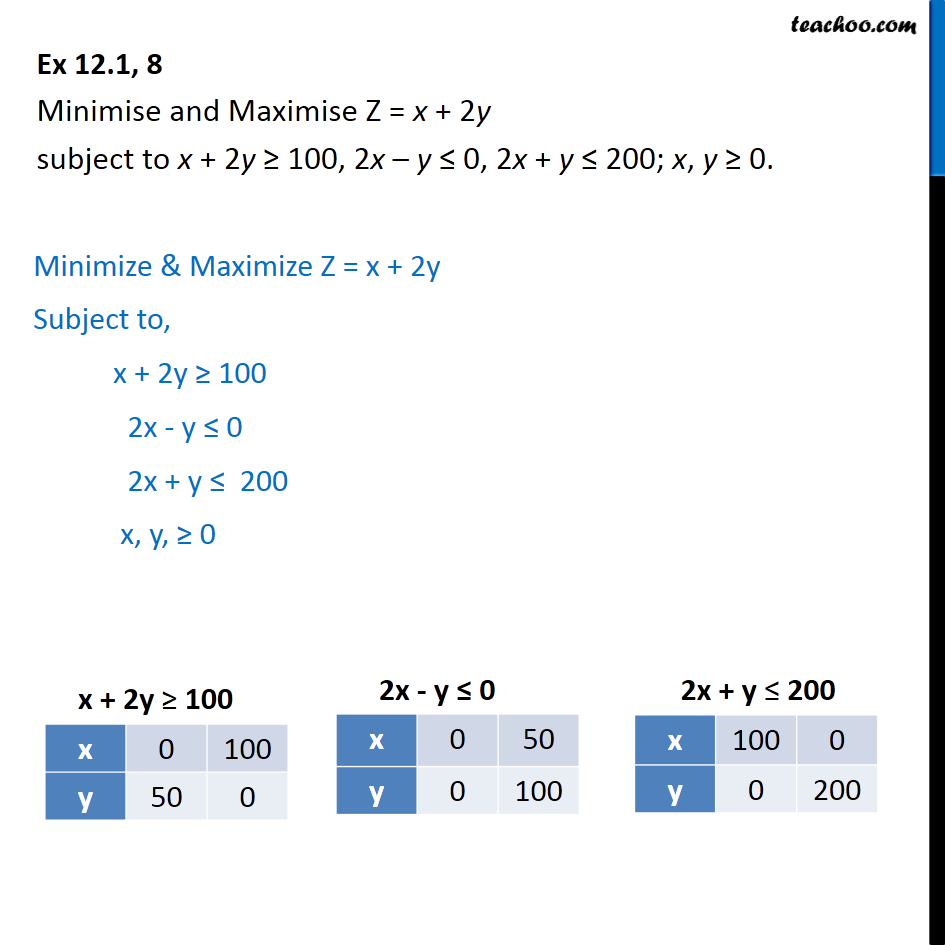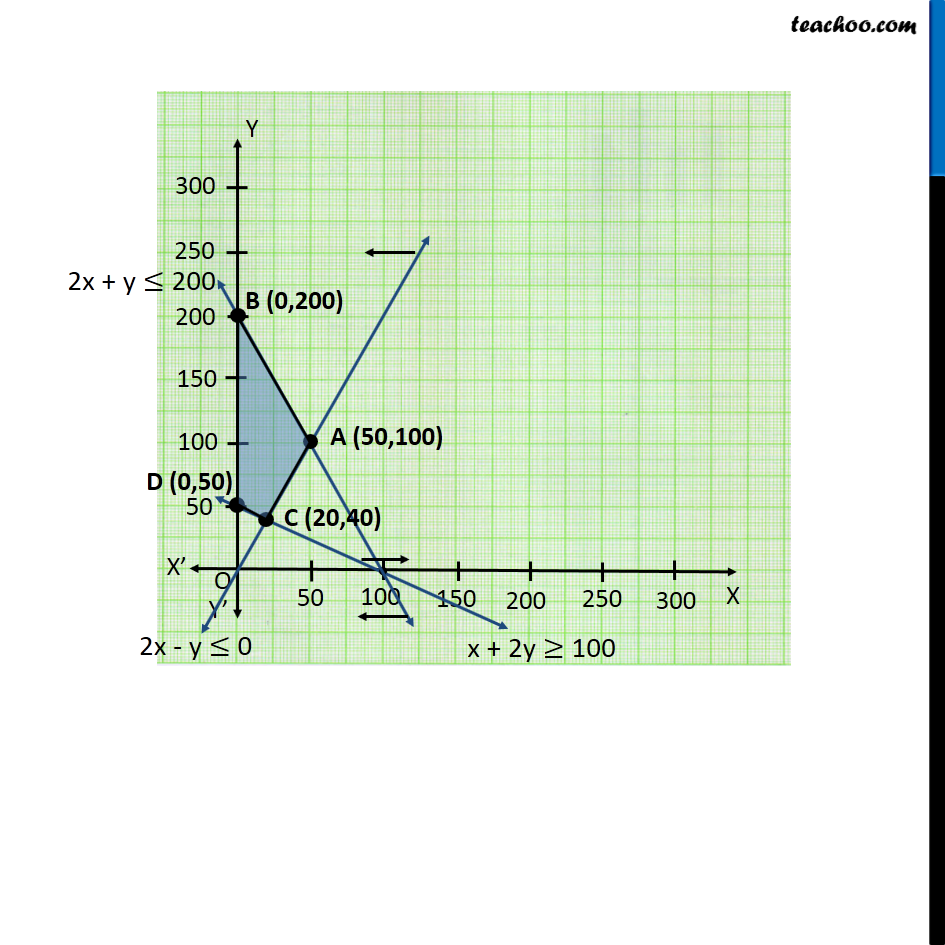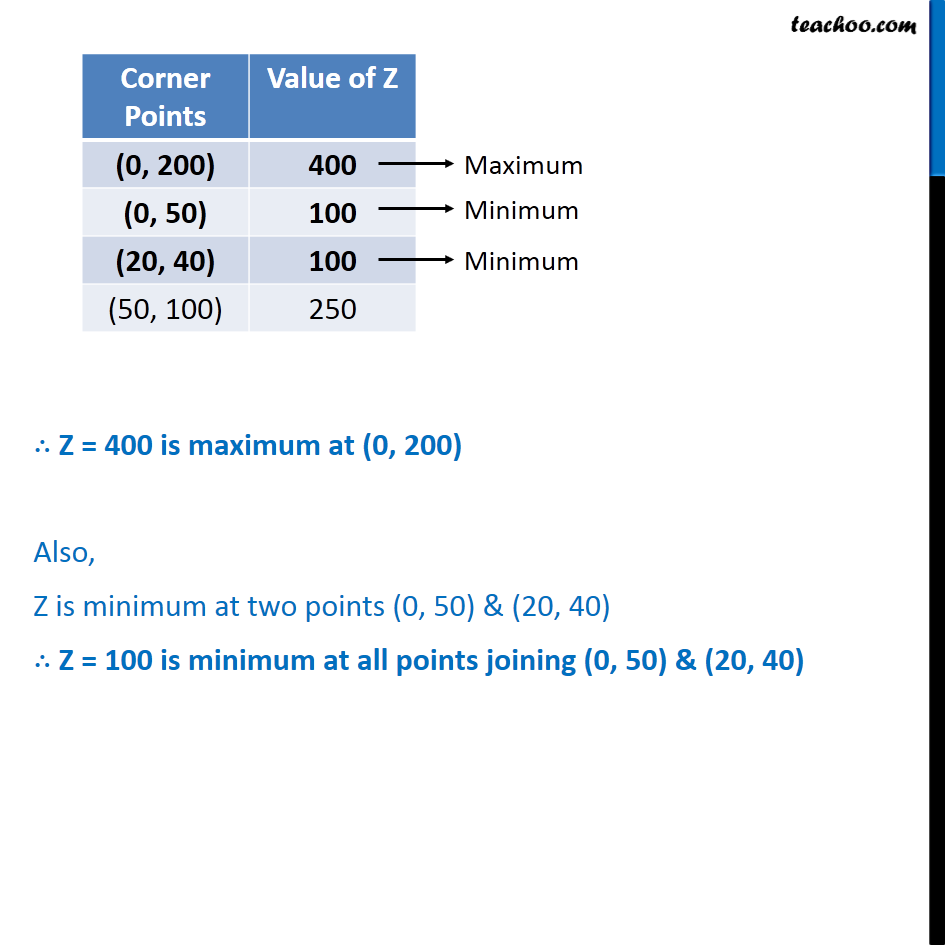Ex 12.1

Chapter 12 Class 12 Linear Programming
Serial order wiseLearn in your speed, with individual attention - Teachoo Maths 1-on-1 Class

### Transcript

Ex 12.1, 8 Minimise and Maximise Z = x + 2y subject to x + 2y ≥ 100, 2x – y ≤ 0, 2x + y ≤ 200; x, y ≥ 0. Minimize & Maximize Z = x + 2y Subject to, x + 2y ≥ 100 2x - y ≤ 0 2x + y ≤ 200 x, y, ≥ 0 ∴ Z = 400 is maximum at (0, 200) Also, Z is minimum at two points (0, 50) & (20, 40) ∴ Z = 100 is minimum at all points joining (0, 50) & (20, 40)